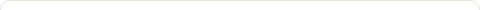# WBM1998: INVESTMENT RISK & RETURN

Posted on: May 24, 2020

THE WBM POSTS ARE MY LOST WORKS FOUND IN THE WAY BACK MACHINE

IS THE RISK-RETURN RELATIONSHIP IN FINANCE AN ARTIFACT?

1. Modern financial theory pre-supposes a rational risk averse behavioral pattern of investors that forms the basis of portfolio theory and the theory and models for the pricing or risky assets. The actual risk and return metrics for stock market investments are defined and computed as follows.
2. Mean stock returns” is the arithmetic average of the series k(i)=(P(i)+Div(i))/P(i-1)-1 where P is the price and Div represents any cash distributions received. In most instances stock dividend yields are very small and so most index yields are estimated simply on the basis of price appreciation as k=P2/P1-1.
3. Risk” is computed as the standard deviation of the k(i) series returns. In case of portfolio effects some of the standard deviation may cancel and this of course leads to portfolio theory. But the residual portfolio risk is really a portion of the standard deviation. Therefore the underlying relationship is that between mean returns and standard deviation. Here we investigate this relationship when returns are generated by price changes alone.
4. Because of the way they are defined both returns and risk are generated by price movements and therefore the two metrics are mathematically related. Some numerical examples expose the nature of the relationship. First consider two stocks U1 and U2 each with a current price of \$100. In the following periods the price of U1 rises steadily at \$1 per period and that of U2 rises at \$2 per period. After 49 periods we compute the mean returns and standard deviation and find that U2 offers higher returns at higher risk. But this relationship was arrived at purely algebraically without any behavioral assumptions. To underscore this point consider now two stocks D1 and D2 that decline in price at \$1 per period and \$2 per period repsectively. After 49 periods we find that D1 offers a higher return at lower risk. Once again the relationship is imposed by mathematics and not by human behavior.
5. However, long run stock market trend are upward with only brief bear periods. Therefore, the data will appear to show the generally assumed high risk – high returns pattern because of a purely mathematical trend effect. There is no underlying theory or philosophy, just math.
6. I demonstrate this historical relationship with monthly S&P500; data from 1972 to 1992. The historical series is shown in Figure 1. The annual market volatility is shown in Figure 2. Finally, in Figure 3 we see the positive risk-return relationship. But when we reverse the S&P500; series as shown in Figure 4 we compute the volatilities shown in Figure 5 and the downward sloping risk return relationship shown in Figure 6.
7. Now consider stocks V1, V2, V3, three stocks with a mean price of \$100 but with different price volatilities. The price of V1 fluctuates by \$1 per period about the mean and that of V2 by \$2, and V3 by \$3. The average price of all three stocks (regardless of the number of periods) is \$100 and the mean returns and standard deviations are higher for V3 than for V2 and higher for V2 than for V1. Once again we are able to generate the positive risk-return relationships of finance purely mathematically.
8. The V1,V2,V3 relationship exists because of the way returns are defined and computed. Returns are higher on an uptick than on an equal price downtick because the divisor is higher in the downtick. And the greater the volatility the greater this odd effect of returns; and hence the high risk high return relationship. The risk and return values of stocks U1, U2, D1, D2, V1, V2, and V3 are shown in Table 1.
9. Since these relationships exist independent of behavior, the relevance of the empirical data to behavioral theory is uncertain. At the least, the data used to test the fundamental question of whether standard deviation risk is priced must first be partitioned to remove the mathematical effects inherent in the data.
10. The volatility effect shown in V1, V2, and V3 may be reduced by choosing a returns period that is significantly larger than the standard deviation period. For example we might compute returns as year to year price changes and standard deviation as month to month price changes. This is what I have done in Figures 1 thru 6 and as we can see the trend effect still persists.
11. An alternate approach might be to re-define “returns” to remove the hysteresis effect in returns in equal up-tick and down-tick movements. Ideally we would like to have risk and return metrics that are independent of each other. Only if the metrics are independent may we ascribe observed relationships to investor behavior; but we do not see this independence in the data presented below.

Table 1: Results of risk and return computations
Stock Risk Returns

 Stock V1 2.02% 0.02% Stock V2 4.04% 0.08% Stock V3 6.07% 0.18% Stock U1 0.09% 0.81% Stock U2 0.27% 1.39% Stock D1 0.27% -1.37% Stock D2 8.90% -7.25%

FIGURE 1: THE S&P500 INDEX SERIESFIGURE 2: THE VOLATILITY OF THE S&P500 INDEX SERIESFIGURE 3: RISK AND RETURN IN THE S&P500 INDEX SERIESFIGURE 4: THE INVERTED S&P500 SERIESFIGURE 5: VOLATILITY IN THE INVERTED SERIESFIGURE 6: RISK AND RETURN IN THE INVERTED SERIES### 3 Responses to "WBM1998: INVESTMENT RISK & RETURN"Reblogged this on uwerolandgross.Volatility is risk, but risk is no5 limited to volatility.Volatility is risk, but risk is not limited to volatility.• chaamjamal: Thank you for this very intersting comment
• chaamjamal: https://tambonthongchai.com/2021/05/10/the-climate-sensitivity-issue/
• chaamjamal: ONE CAN ALWAYS USE THE CLIMATE SENSITIVITY NEEDED TO FIT A CURVE BUT THE REALITY IS THAT THE INDEPENDENT ASSESSMENTS OF CLIMATE SENSITIVITY SHOWS SUC